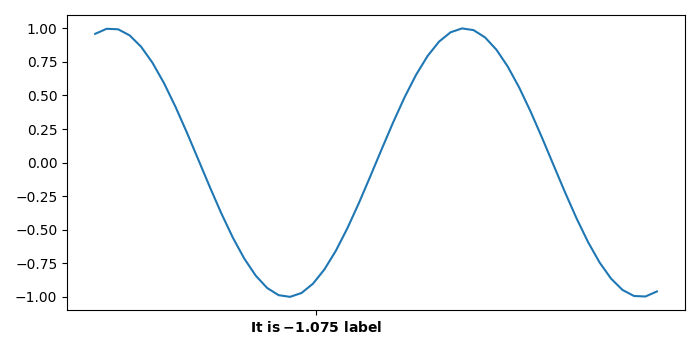# How to append a single labeled tick to X-axis using matplotlib?

Tp append a single labeled tick to X-axis using matplotlib, we can take the following steps.

## Steps

• Set the figure size and adjust the padding between and around the subplots.

• Create x and y data points using numpy.

• Plot x and y data points using plot() method.

• Set xticks at a single point.

• Set the tick label for single tick point.

• To display the figure, use Show() method.

## Example

import numpy as np
import matplotlib.pyplot as plt

# Set the figure size
plt.rcParams["figure.figsize"] = [7.00, 3.50]
plt.rcParams["figure.autolayout"] = True

# Create x and y data points
x = np.linspace(-5, 5, 50)
y = np.sin(x)

# Plot x and y data points
fig, ax = plt.subplots(1, 1)
p = ax.plot(x, y)

# Set xticks at a point
ax.set_xticks([-1.075])

# Set xticklabels for the point
ax.set_xticklabels(["$\bf{It\ is -\!1.075\ label}$"])

# Display the plot
plt.show()


## Output

It will produce the following output −